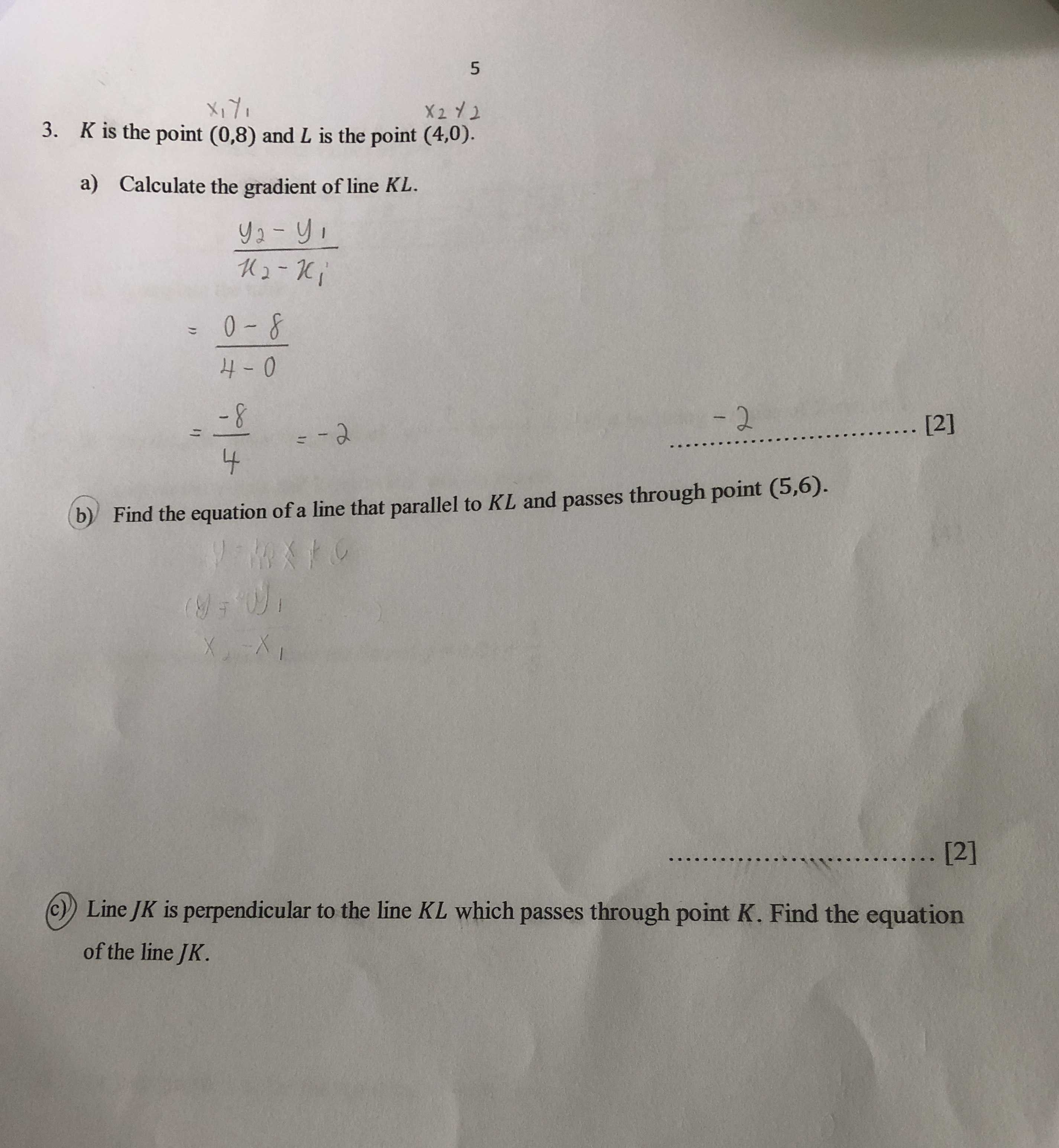### ¿Todavía tienes preguntas de matemáticas?

Pregunte a nuestros tutores expertos
Algebra
Pregunta3. $$K$$ is the point $$( 0,8 )$$ and $$L$$ is the point $$( 4,0 ) .$$

a) Calculate the gradient of line $$K L$$ .

(b) Find the equation of a line that parallel to $$K L$$ and passes through point $$( 5,6 )$$

c)Line $$J K$$ is perpendicular to the line $$K L$$ which passes through point $$K .$$ Find the equation of the line $$J K$$ .

$$- 2$$
$$y= - 2x+ 16$$
$$y= \frac{1}{2} x+ 8$$